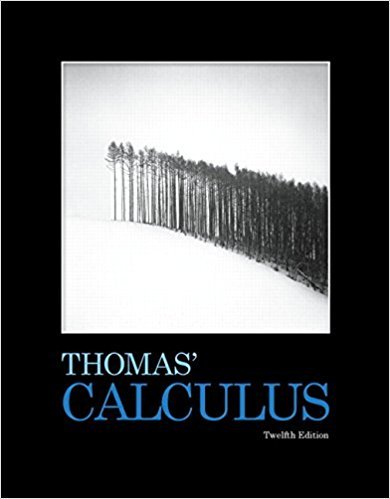×
×

# Solutions for Chapter 1: Functions## Full solutions for Thomas' Calculus | 12th Edition

ISBN: 9780321587992Solutions for Chapter 1: Functions

Solutions for Chapter 1
4 5 0 312 Reviews
17
0
##### ISBN: 9780321587992

Since 68 problems in chapter 1: Functions have been answered, more than 9923 students have viewed full step-by-step solutions from this chapter. This textbook survival guide was created for the textbook: Thomas' Calculus, edition: 12. Thomas' Calculus was written by and is associated to the ISBN: 9780321587992. This expansive textbook survival guide covers the following chapters and their solutions. Chapter 1: Functions includes 68 full step-by-step solutions.

Key Calculus Terms and definitions covered in this textbook

If u = v and w = z , then u + w = v + z

• Boxplot (or box-and-whisker plot)

A graph that displays a five-number summary

• Complex conjugates

Complex numbers a + bi and a - bi

• Convenience sample

A sample that sacrifices randomness for convenience

• DMS measure

The measure of an angle in degrees, minutes, and seconds

• equation of a parabola

(x - h)2 = 4p(y - k) or (y - k)2 = 4p(x - h)

• Factor

In algebra, a quantity being multiplied in a product. In statistics, a potential explanatory variable under study in an experiment, .

• Local maximum

A value ƒ(c) is a local maximum of ƒ if there is an open interval I containing c such that ƒ(x) < ƒ(c) for all values of x in I

• Logarithm

An expression of the form logb x (see Logarithmic function)

• Multiplicative inverse of a real number

The reciprocal of b, or 1/b, b Z 0

• n factorial

For any positive integer n, n factorial is n! = n.(n - 1) . (n - 2) .... .3.2.1; zero factorial is 0! = 1

• Observational study

A process for gathering data from a subset of a population through current or past observations. This differs from an experiment in that no treatment is imposed.

• Reference triangle

For an angle ? in standard position, a reference triangle is a triangle formed by the terminal side of angle ?, the x-axis, and a perpendicular dropped from a point on the terminal side to the x-axis. The angle in a reference triangle at the origin is the reference angle

• Reflection through the origin

x, y and (-x,-y) are reflections of each other through the origin.

• Regression model

An equation found by regression and which can be used to predict unknown values.

• Relevant domain

The portion of the domain applicable to the situation being modeled.

• Slope-intercept form (of a line)

y = mx + b

• Union of two sets A and B

The set of all elements that belong to A or B or both.

• Upper bound for real zeros

A number d is an upper bound for the set of real zeros of ƒ if ƒ(x) ? 0 whenever x > d.

• Vertex of a parabola

The point of intersection of a parabola and its line of symmetry.

×